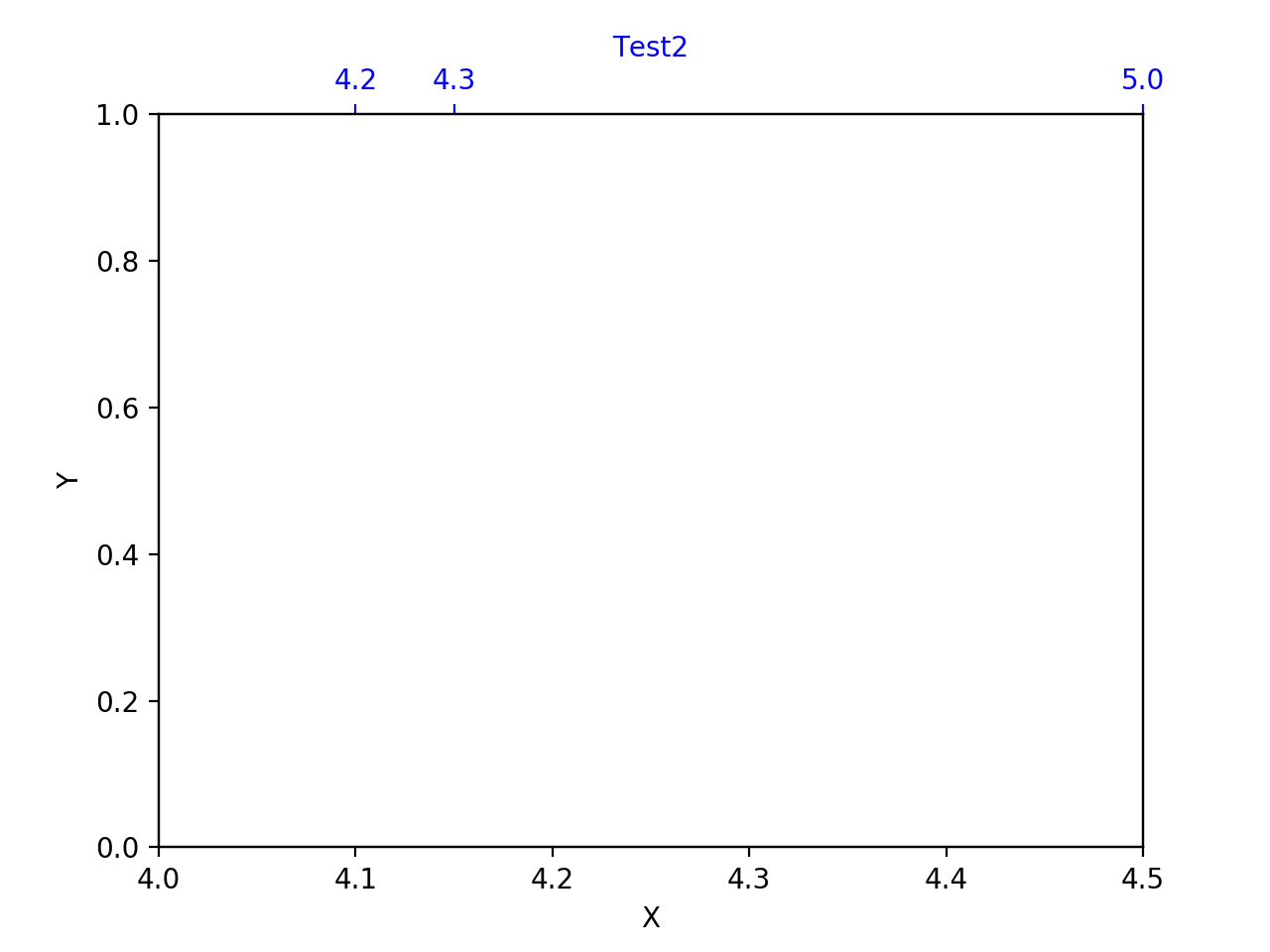# set_ticks() changes view limits of the axis

### Bug report

Bug summary

It seems that calling ax.set_ticks() after the axis range has been defined with ax.set_xlim() will change that range invisibly.

This seems to me like a bit of a trap for unwary plotters.

The example code constructs a plot with two axes meant to be the same, but depending on the value of the ticks set they can end up being different.

Code for reproduction

```import matplotlib.pyplot as plt

filename = "plot.png"

fig, ax1 = plt.subplots()
ax1.set_xlabel("X")
ax1.set_ylabel("Y")
ax1.tick_params('y', colors='k')
ax1.set_ylim([0, 1])

ax2 = ax1.twiny()
ax2.set_ylim([0, 1])

ax2.tick_params(axis="x", colors="blue")
ax2.set_xlabel('Test2', color='b')

xx =[4.2, 4.3, 5.0]
#xx =[4.2, 4.3]    # Try uncommenting this line!

ax1.set_xlim([4.0, 4.5])
ax2.set_xlim([4.0, 4.5])

## This changes xmin, xmax depending on values of xx
## and makes ax2 different to ax1
ax2.xaxis.set_ticks(xx)

plt.savefig(filename)```

Actual outcomeExpected outcome

I would expect the plot to show the two axes (blue and black X axis) agreeing.
Note if I edit the above code to move the set_ticks() call before the set_xlim() call this does happen. But I would not expect the order these are called to matter.

A quick look at the set_ticks() source code shows it auto-expands the axis range!
https://matplotlib.org/3.1.3/_modules/matplotlib/axis.html#Axis.set_ticks

I am not sure of the logic behind this, perhaps it should be reconsidered?

This might be same as issue #8320 ?

Matplotlib version

• Operating system: Mac OS 10.14.6
• Matplotlib version: 3.1.3
• Matplotlib backend (`print(matplotlib.get_backend())`):
• Python version: 3.7.6

Used anacondaFull dicussion on github.com

## 1 possible answer(s) on “set_ticks() changes view limits of the axis”

1. I’m not sure this is something that can be changed. I think the best thing here is to update the docstring of `set_ticks()` to mention that the axis limits are also updated by the method.